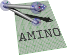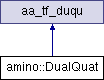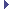amino  1.0-beta2 Lightweight Robot Utility Library
amino::DualQuat Struct Reference

A dual quaternion object. More...

#include <tf.hpp>

Inheritance diagram for amino::DualQuat:## Public Member Functions

DualQuat ()
Construct an identity dual quaternion.

DualQuat (const double *S)
Construct from another dual quaternion.

DualQuat (const struct aa_tf_duqu *S)
Construct from another dual quaternion.

DualQuat (const struct aa_tf_duqu &S)
Construct from another dual quaternion.

DualQuat (const struct aa_tf_qv *S)
Construct from a quaternion-vector.

DualQuat (const struct aa_tf_qv &S)
Construct from a quaternion-vector.

DualQuat (const struct aa_tf_quat *r, const struct aa_tf_vec3 *v)
Construct from a quaternion-vector.

DualQuat (const struct aa_tf_quat &r, const struct aa_tf_vec3 &v)
Construct from a quaternion-vector.

DualQuat (const struct aa_tf_tfmat *T)
Construct from a transformation matrix.

DualQuat (const struct aa_tf_tfmat &T)
Construct from a transformation matrix.

DualQuat (const XAngle &r, const struct aa_tf_vec3 &v)
Construct from a rotation about X and a translation vector.

DualQuat (const YAngle &r, const struct aa_tf_vec3 &v)
Construct from a rotation about Y and a translation vector.

DualQuat (const ZAngle &r, const struct aa_tf_vec3 &v)
Construct from a rotation about Z and a translation vector.

DualQuat (const struct aa_tf_vec3 &v)
Construct from an identity rotation and a translation vector.

DualQuat (const aa_tf_axang &r, const struct aa_tf_vec3 &v)
Construct from an axis-angle rotation and a translation vector.

DualQuat conj ()
Return the conjugate of this.

aa_tf_vec3 translation ()
Return the translation part of a unit dual quaternion.

## Static Public Member Functions

static aa_tf_duqu from_xyzw (double x_real, double y_real, double z_real, double w_real, double x_dual, double y_dual, double z_dual, double w_dual)
Create a dual quaternion from components.

static aa_tf_duqu from_duqu (const double s)
Create a dual quaternion from another dual quaternion.

static aa_tf_duqu from_qv (const double q, const double v)
Create a dual quaternion from a rotation quaternion and a translation vector.

static aa_tf_duqu from_tfmat (const double T)
Create a dual quaternion from a transformation matrix.

static aa_tf_duqu from_xxyz (double theta, double x, double y, double z)
Create a dual quaternion an X axis rotation and translation components.

static aa_tf_duqu from_yxyz (double theta, double x, double y, double z)
Create a dual quaternion an Y axis rotation and translation components.

static aa_tf_duqu from_zxyz (double theta, double x, double y, double z)
Create a dual quaternion an Z axis rotation and translation components.

static aa_tf_duqu from_xyz (double x, double y, double z)
Create a dual quaternion from and identity rotation and translation components.

static aa_tf_duqu from_dx (double *s, double *dx)
Create a dual quaternion derivative from a dual quaternion and the spatial velocity.Public Attributes inherited from aa_tf_duqu
union {
struct {
aa_tf_quat_t   real

aa_tf_quat_t   dual

}

double   data 
data array

};

## Detailed Description

A dual quaternion object.

Definition at line 584 of file tf.hpp.

The documentation for this struct was generated from the following file: